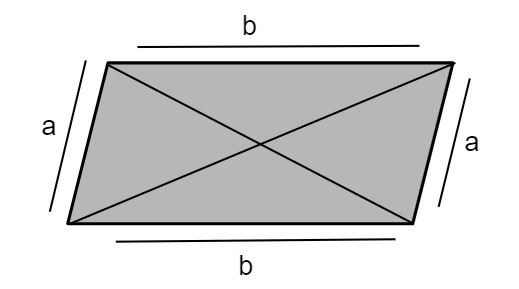# C Program for Circumference of a Parallelogram

We are given with the sides of parallelogram and the task is to generate the circumference of a parallelogram with its given sides and display the result

## What is a Parallelogram?

Parallelogram is a type of quadratic which have −

• Opposite sides parallel
• Opposite angles equal
• Polygon diagonals bisects each other

Shown in the below figure ‘a’ and ‘b’ are the sides of a parallelogram where parallel sides are shown in the figure.Perimeter/Circumference of a parallelogram is defined as

Circumference of Parallelogram = 2(a + b)

= 2 * a + 2 * b

## Example

Input-: a = 23 and b = 12
Output-: Circumference of a parallelogram is : 70.00
Input-: a = 16.2 and b = 24
Output-: Circumference of a parallelogram is : 80.4

## Algorithm

START
Step 1-> Declare function to calculate circumference of parallelogram
float circumference(float a, float b)
return ((2 * a) + (2 * b))
Step 2-> In main()
Declare float a = 23, b = 12
Call circumference(a, b)
STOP

## Example

#include <stdio.h>
//function for circumference of parallelogram
float circumference(float a, float b) {
return ((2 * a) + (2 * b));
}
int main() {
float a = 23, b = 12;
printf("Circumference of a parallelogram is : %.2f", circumference(a, b));
return 0;
}

## Output

Circumference of a parallelogram is : 70.00